These are following important points about circle in geometry :

1. Equation of circle having center at (0, 0) and radius r :
`x2+y2=r2 `
2. Equation of circle having center at (h, k) and radius a :
`(x-h)2+(y-k)2=a2 `
3. Standard equation of circle is x2+y2+2gx+2fy+c=0 where radius=?(g2+f2-c) and center at(-g, -f) and condition is g2+f2-c > = 0
4. If  g2+f2-c=0 then equation represents a point circle having center only (-g, -f).
5. Diametrical form of a circle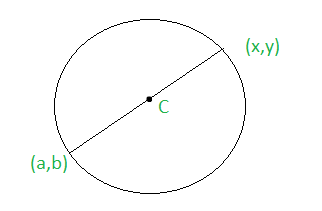Figure – (X-x)(X-a)+(Y-y)(Y-b) = 0

```S1=x12+y12+2gx1+2fy1+c
S2=x22+y22+2gx2+2fy2+c
```
6. Equation of Circle Passing through point of intersection of circles S1=0 and S2=0 is S1+kS2=0 where k is not equal to -1.
7. Equation of circle passing through a point of intersection of circle s=0 and line u=0 is s+ku=0
8. If the circles S1=0 and S2=0 intersect then S1-S2=0 is their common chord.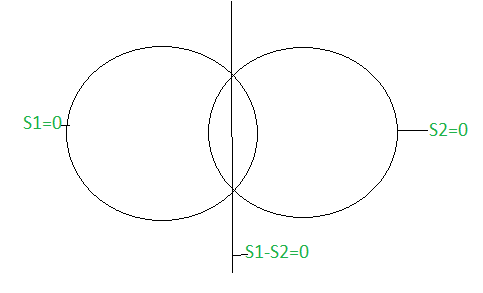9. If two circles S1=0 and S2=0 have internal contact the S1-S2=0 is their internal common tangent.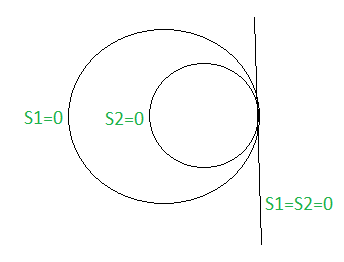10. If Two Circles S1=0 and S2=0 do not intersect then S1-S2=0 is their radial axis.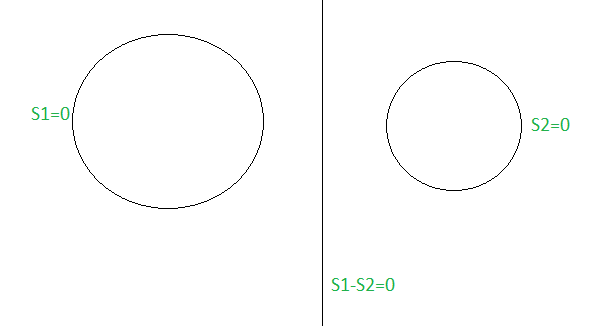11. If Two Circles S1=0 and S2=0 have external contact the S1-S2=0 is their external common tangent.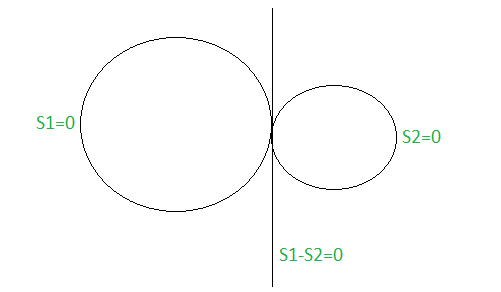Attention reader! Don’t stop learning now. Get hold of all the important CS Theory concepts for SDE interviews with the CS Theory Course at a student-friendly price and become industry ready.

My Personal Notes arrow_drop_upCheck out this Author's contributed articles.

If you like GeeksforGeeks and would like to contribute, you can also write an article using contribute.geeksforgeeks.org or mail your article to contribute@geeksforgeeks.org. See your article appearing on the GeeksforGeeks main page and help other Geeks.

Please Improve this article if you find anything incorrect by clicking on the "Improve Article" button below.

Article Tags :

Be the First to upvote.

Please write to us at contribute@geeksforgeeks.org to report any issue with the above content.